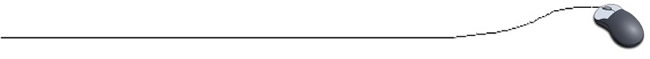One Dimensional Arrays
Large Amounts of Data
An array is a collection of data storage locations, each of which holds the same type of data.  Think of an array as a "box" drawn in memory with a series of subdivisions, called elements, to store the data.  The box below could represent an array of 10 integers.

 7 6 5 8 3 9 2 6 10 2

While this array contains only 10 elements, arrays are traditionally used to handle large amounts of data.  It is important to remember that all of the elements in an array must contain the same type of data

Let's consider a programming situation "with" and "without"
the use of an array:
Without an Array
Memory
Suppose you must calculate the average of 3 integer values:
avg = (score1 + score2 + score3) / 3;

Yes, this will be integer division.
 score1
 score2
 score3
Looking good!  But what if there were 50 scores?  You would need 50 separate variable names with 50 lines of input.  Yeek!
OK!  If you have to deal with a large number of scores, you could use a loop.
int sum = 0;
for ( i = 1; i <= 50; i++ )
{
System.out.print("Enter score");
sum = sum + score;
}
avg = sum / 50.0;
 score
Still looking good!  But what if, after finding the average, you need to find how many scores were above/below the average?  Yeek!  The only score entered by the user that is left in memory is the last one.
With an Array
You can see that dealing with large amounts of data can present potential problems, as shown above.  With an array, however, only one variable name is needed and all entries will remain in the memory for future use.  Here we have an array named scores (one name, not 50 separate names) with 50 cells to hold the entries.  All entries will remain in the memory for future use. (Not all 50 cells are shown in the array below.)
scores
 . . .

scores is the name of a 50 element (cell) array.  The elements are like mailboxes in a large apartment complex.  The "name of the array" is the address of the apartment complex where all of the mailboxes reside.Mantra:  The name of the array is the address of the first element of the array!